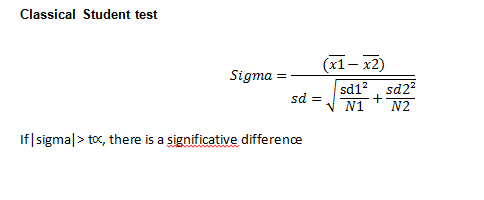Scroll

# DOC : Significance and Column Significativity test formulas

Follow
 Summary This article describes the algorithms used in the significance and column significativity test in askiaanalyse. Applies to askiaanalyse Written for Data processor Keywords significance; column significativity; test; analyse; askiaanalyse

Here is a decision chart to pick your test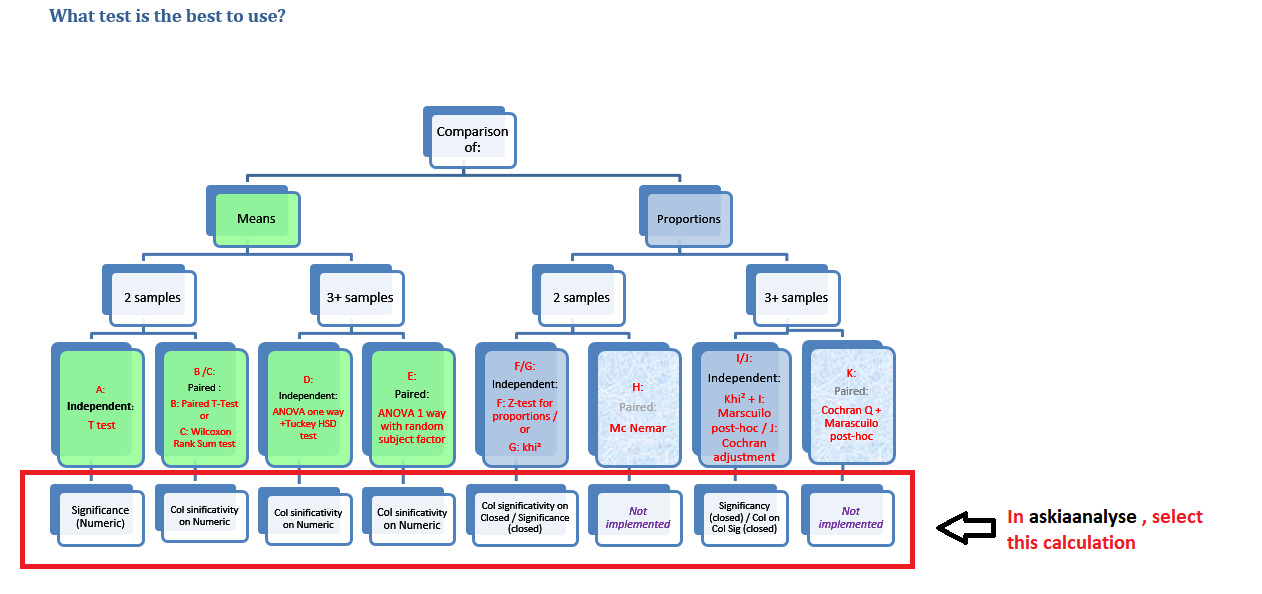Significance test formulas

Independence test (I)

In the results, significant values will be indicated by signs, as follows (if the corresponding display options described above are selected) :

• High significativity : t∝=99%=2,576 "+++" or" ---"
• Normal significativity : t∝=95%=1,96 "++" or "-- "
• Low significativity: t∝=90%=1,65 "+" or "-"

We compare the calculated Sigma to the significativity threshold:
If Sigma > test value, then there is a significative difference.
The sign will indicate if the percentage is significatively decreasing ("-") or increasing ("+").

1. counts when independent (khi²) (I)

```N : Total Sample size

Effind(i,j) = (Total(i) * Total(j) )/N
PrcInd (i,j) = (Effind(i,j) )/N
PrcObs (i,j) = (Observed(i,j) )/N
```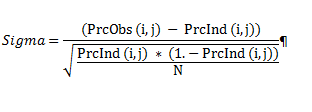2. all other columns/rows (I)

```All other columns(j) All other rows (i)
N1 = Total(j) N1 = Total(i)
N2 = N - N1
```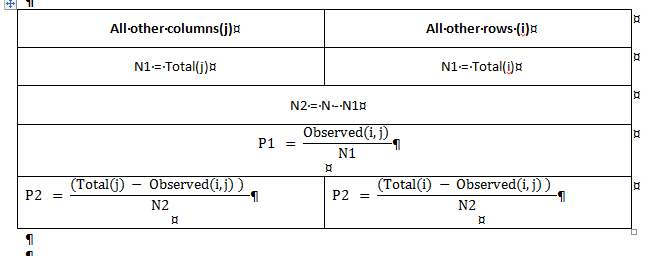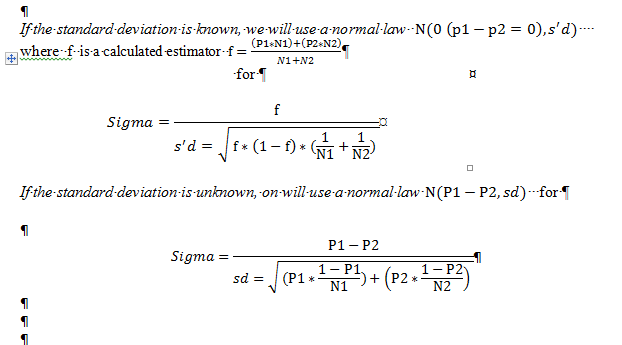Column Significativity test formulas

You will have letters in the table with different size following the significativity threshold (a, A, A+)

• High significativity : t∝=99%=2,576 "A+"
• Normal significativity : t∝=95%=1,96 "A"
• Low significativity: t∝=90%=1,65 "a"

We compare the calculated Sigma to the significativity threshold:

If |Sigma| > t∝, then there is a significative difference.

The letter “A” will indicate which cell is different from the other cell in column or in row. The letter could be displayed on the column/row with:

• The highest value
• The first column
• The previous column
• Both column

Frequency comparison test

```N1 : Sample 1 size
N2 : Sample 2 size
Eff1 : Count n the cell in N1 (weighted)Eff2 : Count in the cell in N2 (weighted) No1: Sum(Eff1-¯x1)² / Sum (Eff1²) => Efficiency base in N1No2: Sum(Eff2-¯x2)² / Sum (Eff2²) => Efficiency base in N2
¯x1=Average of weights in the N1
¯x2=Average of weights in the N2

dP1 : Percentage 1 => Eff1/N1
dP2 : Percentage 2=> Eff2/ N2
dFo : Estimated percentage => (eff1+eff2) / (N1+N2)
```

Classical Student Test (F)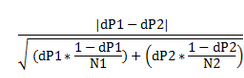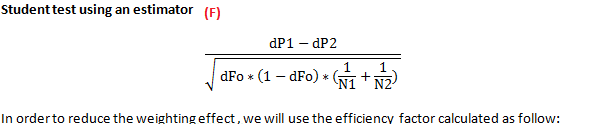the ‘Unequal Weighting Effect’ (UWE)
Leslie Kish has analysed the effect of unequal weights in the accuracy of estimations through the ‘Unequal Weighting Effect’ (UWE). (Kish L., Weighting for Unequal Pi, Journal of Official Statistics, Vol. 8, N°2, 1992, pp. 183-200.)

If we have wi the weight per individual (weighting sample factor) and n the global size sample, the factor (UWE) of the variance increase of weight per individual, is: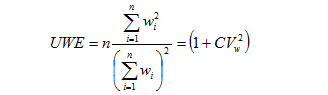The relative increase of variance is equal to 1+ the squared of the weighting variance factor (CVw²).
To include this UWE in the calculation, we can replace the total count of individuals n by n0 in the the classical formula denominator. n0 is a fictive number which includes a under /over representation of categories in the sample weighted,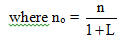This new base is named Efficiency base and the ratio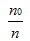, efficiency index.

The efficiency base is calculated as follow:

```No =No1+No2
```

The efficiency coefficient =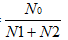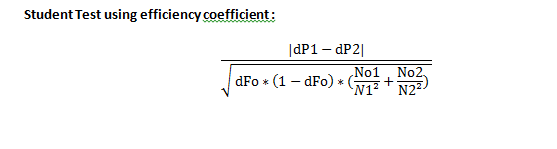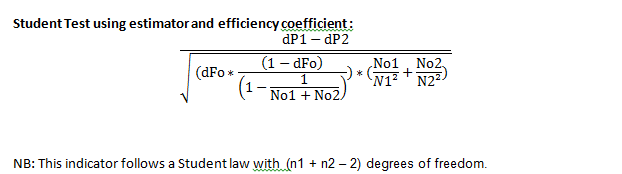Mean comparison

```N1 : Sample 1 size
N2 : Sample 2 size
¯x1=Mean 1
¯x2=Mean 2
Sd1= Standard deviation in N1
Sd2= Standard deviation in N2
t∝=90%=1,65
t∝=95%=1,96
t∝=99%=2,576

Sigma follows a normal law N((¯x1-¯x2=0),sd)
```